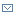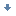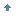• 博客访问： 4230372
• 博文数量： 473
• 博客积分： 12019
• 博客等级： 上将
• 技术积分： 6515
• 用 户 组： 普通用户
• 注册时间： 2005-08-01 16:46

2020年（30）

2019年（4）

2018年（10）

2017年（5）

2016年（2）

2015年（4）

2014年（4）

2013年（16）

2012年（47）

2011年（65）

2010年（46）

2009年（34）

2008年（52）

2007年（52）

2006年（80）

2005年（22）

2006-02-21 13:05:02

# 将信号用作 Linux 调试工具文档选项将此页作为电子邮件发送未显示需要 JavaScript 的文档选项

 对此页的评价帮助我们改进这些内容

Madhavan Srinivasan , 开发人员, PowerPC Tools Development, IBM

2005 年 12 月 19 日

 `````` #include #include main() { signal(SIGINT,SIG_IGN); while(1) printf("You can't kill me with SIGINT anymore, dude\n"); return 0; } ``````

• 默认操作，其中内核会对信号进行处理，并根据信号的不同执行适当的操作。每个信号在内核中都有自己的信号处理程序；信号处理程序的默认行为是终止进程。
• 执行用户定义的操作，此时这个信号由一个用户定义的信号处理程序来处理。回页首

 `````` struct sigaction mysig_act; mysig_act.sa_flags = SA_SIGINFO; mysig_act.sa_sigaction = (void *)mysig_handler; if(sigaction (,&mysig_act,(struct sigaction *)NULL)) { printf("Sigaction returned error = %d\n", errno); exit(0); } ``````

`sigaction` 系统调用需要使用 3 个参数：

• 信号编号
• 指向新 `sigaction` 结构体的指针
• 指向旧 `sigaction` 结构体的指针

`sigaction` 结构体的定义如清单 3 所示：

 `````` struct sigaction { void (*sa_handler)(int); /* func pointer */ void (*sa_sigaction)(int, siginfo_t *, void *); /*func pointer */ sigset_t sa_mask; int sa_flags; void (*sa_restorer)(void); } ``````

• 信号编号
• 信号信息
• 硬件上下文的快照

`mysig_handler` 是在接收到信号时要调用的处理函数。`mysig_act` 是一个 `sigaction` 结构体，其中包含了所有的信息。

 `````` #define PPC_REG unsigned long struct pt_regs { PPC_REG gpr; PPC_REG nip; PPC_REG msr; PPC_REG orig_gpr3; /* Used for restarting system calls */ PPC_REG ctr; PPC_REG link; PPC_REG xer; PPC_REG ccr; PPC_REG softe; /* Soft enabled/disabled */ PPC_REG trap; /* Reason for being here */ PPC_REG dar; /* Fault registers */ PPC_REG dsisr; PPC_REG result; /* Result of a system call */ }; ``````

 `````` #include #include #include #include static void myhandler (unsigned int sn , siginfo_t si , struct ucontext *sc) { unsigned int mnip; int i; printf(" signal number = %d, signal errno = %d, signal code = %d\n", si.si_signo,si.si_errno,si.si_code); printf(" senders' pid = %x, sender's uid = %d, \n",si.si_pid,si.si_uid); } main() { struct sigaction s; s.sa_flags = SA_SIGINFO; s.sa_sigaction = (void *)myhandler; if(sigaction (SIGTERM,&s,(struct sigaction *)NULL)) { printf("Sigaction returned error = %d\n", errno); exit(0); } while(1); return 0; } ``````

 `````` > ./fin &  7375 > ps -ef | grep 7375 maddy 7375 7063 90 16:51 pts/0 00:00:24 ./fin maddy 7377 7063 0 16:52 pts/0 00:00:00 grep 7375 > kill 7375 signal number = 15, signal errno = 0, signal code = 0 senders' pid = 7063, sender's uid = 1001, > kill -9 7375 > ps -ef | grep 7375 maddy 7379 7063 0 16:52 pts/0 00:00:00 grep 7375 + Killed ./fin ``````

 `````` #include #include #include #include static void myhandler (unsigned int sn , siginfo_t si ,\ struct ucontext *sc) { unsigned int mnip; int i,j; printf(" Signal number = %d, Signal errno = %d\n" ,si.si_signo,si.si_errno); switch(si.si_code) { case 1: printf(" SI code = %d (Illegal opcode)\n",si.si_code); break; case 2: printf(" SI code = %d (Illegal operand)\n",si.si_code); break; case 3: printf(" SI code = %d (Illegal addressing mode)\n", si.si_code); break; case 4: printf(" SI code = %d (Illegal trap)\n",si.si_code); break; case 5: printf(" SI code = %d (Privileged opcode)\n",si.si_code); break; case 6: printf(" SI code = %d (Privileged register)\n",si.si_code); break; case 7: printf(" SI code = %d (Coprocessor error)\n",si.si_code); break; case 8: printf(" SI code = %d (Internal stack error)\n",si.si_code); break; default: printf("SI code = %d (Unknown SI Code)\n",si.si_code); break; } printf(" Machine State Register = %x \n", (((struct pt_regs *)((&(sc->uc_mcontext))->regs))->msr)); printf(" Link register pointing to location = 0x%x, \ Opcode at the location = 0x%x \n", (((struct pt_regs *)((&(sc->uc_mcontext))->regs))->link), *(unsigned int *) \ (((struct pt_regs *)((&(sc->uc_mcontext))->regs))->link)); for(i=20,j=5;i>0;i-=4,j--) printf(" Op-Code [nip - %d] = 0x%x at address = 0x%x \n" ,j,*(unsigned int *)(si.si_addr - i) ,(si.si_addr - i) ); printf(" Failed Op-code = 0x%x at address = 0x%x \n", *(unsigned int*)(si.si_addr), (si.si_addr)); printf(" Op-Code [nip + 1] = 0x%x at address = 0x%x \n", *(unsigned int *)(si.si_addr + 4), (si.si_addr + 4)); (((struct pt_regs *)((&(sc->uc_mcontext))->regs))->nip) += 4; } my() { __asm__ volatile ("add 4,5,6 \n\t":); __asm__ volatile ("add 7,8,9 \n\t":); __asm__ volatile ("mfmsr 3 \n\t":); __asm__ volatile ("add 4,5,6 \n\t":); __asm__ volatile ("add 7,8,9 \n\t":); } main() { struct sigaction s; s.sa_flags = SA_SIGINFO; s.sa_sigaction = (void *)myhandler; if(sigaction (SIGILL,&s,(struct sigaction *)NULL)) { printf("Sigaction returned error = %d\n", errno); exit(0); } my(); return 0; } ``````

 `````` > ./mysigill Signal number = 4, Signal errno = 0 SI code = 5 (Privileged opcode) Machine State Register = 4d032 Link register pointing to location = 0x10000830, Opcode at the location = 0x38000000 Op-Code [nip - 5] = 0x9421ffe0 at address = 0x10000788 Op-Code [nip - 4] = 0x93e1001c at address = 0x1000078c Op-Code [nip - 3] = 0x7c3f0b78 at address = 0x10000790 Op-Code [nip - 2] = 0x7c853214 at address = 0x10000794 Op-Code [nip - 1] = 0x7ce84a14 at address = 0x10000798 Failed Op-code = 0x7c6000a6 at address = 0x1000079c Op-Code [nip + 1] = 0x7c853214 at address = 0x100007a0 ``````

 `````` > objdump -S mysigill >> /tmp/mdmp ``````

/tmp/mdmp 文件中保存了可执行文件 mysigill 执行 objdump 之后的结果。首先，查找出错的操作码/指令。在本例中，出错的操作码是 7c6000a6。

 `````` 10000788 : 10000788: 94 21 ff e0 stwu r1,-32(r1) 1000078c: 93 e1 00 1c stw r31,28(r1) 10000790: 7c 3f 0b 78 mr r31,r1 10000794: 7c 85 32 14 add r4,r5,r6 10000798: 7c e8 4a 14 add r7,r8,r9 1000079c: 7c 60 00 a6 mfmsr r3 <== Bingo!!! 100007a0: 7c 85 32 14 add r4,r5,r6 100007a4: 7c e8 4a 14 add r7,r8,r9 100007a8: 7c 03 03 78 mr r3,r0 100007ac: 81 61 00 00 lwz r11,0(r1) 100007b0: 83 eb ff fc lwz r31,-4(r11) 100007b4: 7d 61 5b 78 mr r1,r11 100007b8: 4e 80 00 20 blr ``````

 `````` #include #include #include #include #include #include static void seghandler (unsigned int sn , siginfo_t si , \ struct ucontext *sc) { unsigned int mnip; int i; mnip=*(unsigned int *)(((struct pt_regs *) \ ((&(sc->uc_mcontext))->regs))->nip); printf(" Signal number = %d, Signal errno = %d\n", si.si_signo,si.si_errno); switch(si.si_code) { case 1: printf(" SI code = %d (Address not mapped to object)\n", si.si_code); break; case 2: printf(" SI code = %d (Invalid permissions for \ mapped object)\n",si.si_code); break; default: printf("SI code = %d (Unknown SI Code)\n",si.si_code); break; } printf(" Intruction pointer = %x \n",mnip); printf(" Fault addr = 0x%x \n",si.si_addr); printf(" dar = 0x%x \n", (((struct pt_regs *)((&(sc->uc_mcontext))->regs))->dar)); printf(" trap = 0x%x \n", (((struct pt_regs *)((&(sc->uc_mcontext))->regs))->trap)); printf(" Op-Code [nip - 4] = 0x%x at address = 0x%x \n", *(unsigned int *)\ (((struct pt_regs *)((&(sc->uc_mcontext))->regs))->nip-4), (((struct pt_regs *)((&(sc->uc_mcontext))->regs))->nip-4) ); printf(" Failed Op-code = 0x%x at address = 0x%x \n", *(unsigned int *)\ (((struct pt_regs *)((&(sc->uc_mcontext))->regs))->nip), (((struct pt_regs *)((&(sc->uc_mcontext))->regs))->nip)); printf(" Op-Code [nip + 1] = 0x%x at address = 0x%x \n", *(unsigned int *) \ (((struct pt_regs *)((&(sc->uc_mcontext))->regs))->nip+4), (((struct pt_regs *)((&(sc->uc_mcontext))->regs))->nip + 4)); printf("***GPR values are the time of fault*** \n"); for (i=0;i<11;i++) printf(" Gpr[%d] = 0x%x \n",i, \ (((struct pt_regs *)((&(sc->uc_mcontext))->regs))->gpr[i])); (((struct pt_regs *)((&(sc->uc_mcontext))->regs))->nip)+=4; } main() { struct sigaction m; char *p,*q, arr[]="Ma"; q=arr; m.sa_flags = SA_SIGINFO; m.sa_sigaction = (void *)seghandler; sigaction (SIGSEGV,&m,(struct sigaction *)NULL); *p++ = *q++; return 0; } ``````

 `````` > ./sigsegv Signal number = 11, Signal errno = 0 SI code = 1 (Address not mapped to object) Intruction pointer = 98080000 Fault addr = 0x0 dar = 0x0 trap = 0x300 Op-Code [nip - 4] = 0x88090000 at address = 0x10000760 Failed Op-code = 0x98080000 at address = 0x10000764 Op-Code [nip + 1] = 0x396b0001 at address = 0x10000768 ***GPR values are the time of fault*** Gpr = 0x4d Gpr = 0xffffe070 Gpr = 0x4001ee20 Gpr = 0x0 Gpr = 0xffffdf30 Gpr = 0x0 Gpr = 0xffffe110 Gpr = 0xffffe114 Gpr = 0x0 Gpr = 0xffffe120 Gpr = 0x0 ``````

 `````` 10000744: 48 01 07 21 bl 10010e64 <__bss_start+0x48> *p++ = *q++; 10000748: 38 df 00 a0 addi r6,r31,160 1000074c: 81 46 00 00 lwz r10,0(r6) 10000750: 38 ff 00 a4 addi r7,r31,164 10000754: 81 67 00 00 lwz r11,0(r7) 10000758: 7d 48 53 78 mr r8,r10 1000075c: 7d 69 5b 78 mr r9,r11 10000760: 88 09 00 00 lbz r0,0(r9) 10000764: 98 08 00 00 stb r0,0(r8) <==Failed instruction 10000768: 39 6b 00 01 addi r11,r11,1 1000076c: 91 67 00 00 stw r11,0(r7) 10000770: 39 4a 00 01 addi r10,r10,1 10000774: 91 46 00 00 stw r10,0(r6) return 0; 10000778: 38 00 00 00 li r0,0 } ``````回页首

• 下载 KGDB，这是 Linux 内核的一个源代码级的调试器，可以与 GDB 一起使用对内核进行调试。

• 定购免费的 SEK for Linux，共有两张 DVD，包括最新的 IBM for Linux 的试用版软件以及 DB2®、Lotus®、Rational®、Tivoli® 和 WebSphere®。

• 在您的下一个开发项目中使用 IBM 试用版软件，可以从 developerWorks 上直接下载。回页首Madhavan Srinivasan 获得了印度 Madras 大学的电力电子工程学士学位。他从 2003 年 11 月开始为印度的 IBM Global Services (Software Labs) 工作。作为 IBM PowerPC Tools Development Team 小组的一员，他的主要工作是为 Linux 和 AIX 上的 PowerPC 服务处理器设计和开发诊断工具。他涉猎的其他领域还包括 PowerPC 体系架构和操作系统内幕。